Latest Banking jobs   »

# Reasoning Quiz For ECGC PO 2023 -15th May

Directions (1-5): Study the information carefully and answer the questions given below.
Nine boxes are placed one above another in a stack. Four boxes are placed between box F and box H. More than Four boxes are placed above the box H. Two boxes are placed between box B and box C which is placed above box H. There are three boxes placed between box E and box D which is placed just above box F. More than three boxes are placed between box C and box A which is placed above box B. More than two boxes are placed between box G and box K which is not placed above box C.

Q1. Which of the following box is placed just above box B?
(a) G
(b) H
(c) A
(d) F
(e) None of these

Q2. Which of the following box is placed in the bottom of the stack?
(a) B
(b) K
(c) H
(d) C
(e) None of these

Q3. How Many boxes are placed between box A and Box H?
(a) Four
(b) Three
(c) More than four
(d) One
(e) None

Q4. Which of the following statement is false about box G?
(a) Box G is placed just above box E
(b) Not more than two boxes are placed between box A and G
(c) One box is placed between C and G
(d) More than three boxes are placed above box G
(e) Both (b) and (d)

Q5. Which of the following box is placed just below box C?
(a) D
(b) H
(c) G
(d) A
(e) None of these

Directions (6-10): In these questions, relationship between different elements is show in the statements. The statements are followed by conclusions. Study the conclusions based on the given statements and select the appropriate answer: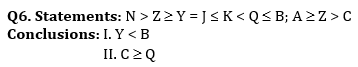(a) Both conclusions I and II are true
(b) Either conclusion I or II is true
(c) Only conclusion I is true
(d) Neither conclusions I nor II is true
(e) Only conclusion II is true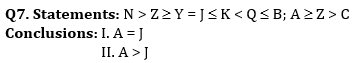(a) Both conclusions I and II are true
(b) Only conclusion II is true
(c) Neither conclusion I nor II is true
(d) Either conclusions I or II is true
(e) Only conclusions I is true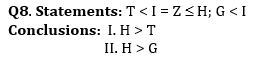(a) Both conclusion I and II are true
(b) Either conclusion I or II is true
(c) Neither conclusion I nor II is true
(d) Only conclusion I is true
(e) Only conclusion II is true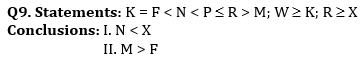(a) Only conclusion II is true
(b) Either conclusion I or II is true
(c) Both conclusion I and II are true
(d) Neither conclusion I nor II is true
(e) Only conclusion I is true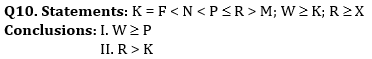(a) Neither conclusion I nor II is true
(b) Both conclusions I and II are true
(c) Only conclusion II is true
(d) Either conclusion I or II is true
(e) Only conclusion I is true

Solutions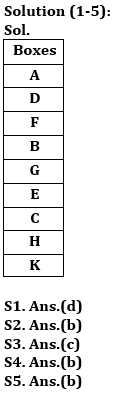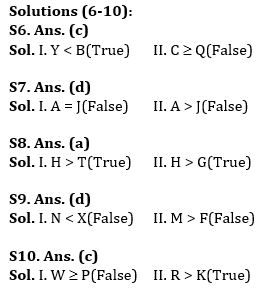## FAQs

### What is the total duration of ECGC PO for objective?

The duration of ECGC PO for objective is 140 minutes

#### Congratulations!Union Budget 2023-24: Free PDF